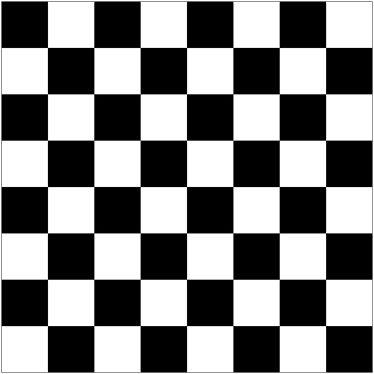# Render a checkerboard

## Part 1

Using the below code as a starting point, create a function called `createSquare` that takes 4 arguments: `x`, `y`, `size`, and `colour`, such that `createSquare(10, 10, 50, "white")` draws a white square 50 pixels in height and width with its top-left corner at the coordinate (x, y).

``````from tkinter import *

c = Canvas(Tk(), width=500, height=500)
c.pack()

c.create_rectangle(10, 10, 60, 60, fill = "white")

mainloop()
``````

## Part 2

Using nested loops, draw a grid of 8x8 squares. Then, make the colour of these squares alternate such that you render a checkerboard pattern like the one below.## Extra

• Extend your `createSquares` function such that it takes a column and row index as an input (from 0 to 7 inclusive) instead of pixel coordinates
• If you have used an iterative approach, use modular arithmetic instead. Recall that `x % 2 == 0` whenever `x` is an even number. Further, `(row + column) % 2 == 0` is true for all black squares where `row` and `column` are its row and column indices (from 0 to 7).